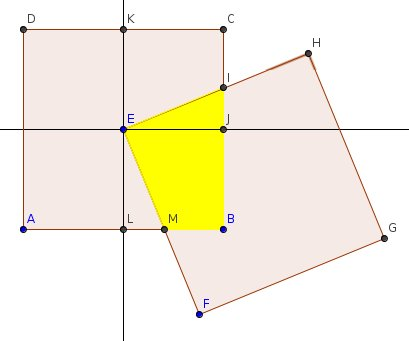### Square deal: a solution

On Futility Closet, Greg Ross proposed an interesting puzzle that i reproduce in the following picture, using GeoGebra:The result of the quest (calculate yellow area, or $A_{EIBM}$) is 4 inch2. Why?
First of all we must proof the congruence between $T_{AEIBM}$ and $T_{EKCJ}$, who is a square's quarter, and so $A_{EKCJ} = 4$.
First of all I proof the congruence between $T_{EJI}$ and $T_{ELM}$

1) $\widehat{LEM} = \beta$ (definition)
2) $\widehat{IJE} = \pi = \widehat{ELM}$
3) $\widehat{IEL} = \pi + \beta = \widehat{LEJ} + \widehat{IEJ} = \pi + \widehat{IEJ}$ (using 2 and 3)
4) So $\widehat{IEJ} = \beta = \widehat{LEM}$
5) $\widehat{IJE} = \pi = \widehat{MLE}$
6) $EJ = EL$ (construction)
Following (4), (5), (6) (according to Euclide) $T_{EJI} = T_{ELM}$

The conclusion is very simple:
$S_{EKCJ} = S_{EJBL}$ for construction.
Now, some calculation:
$A_{EKCI} = A_{EKCJ} - A_{EIJ} = A_{EJBL} - A_{ELM} = A_{EJBM}$
So we can calculate the requested area:
$A_{EJI} + A_{EJBM} = A_{EJI} + A_{EKCI} = 4$